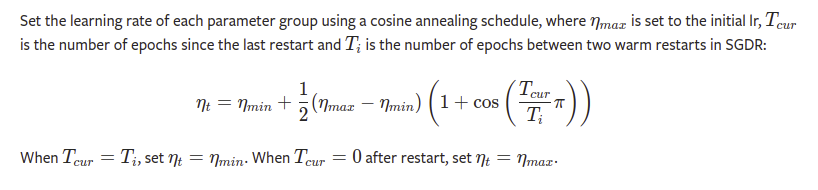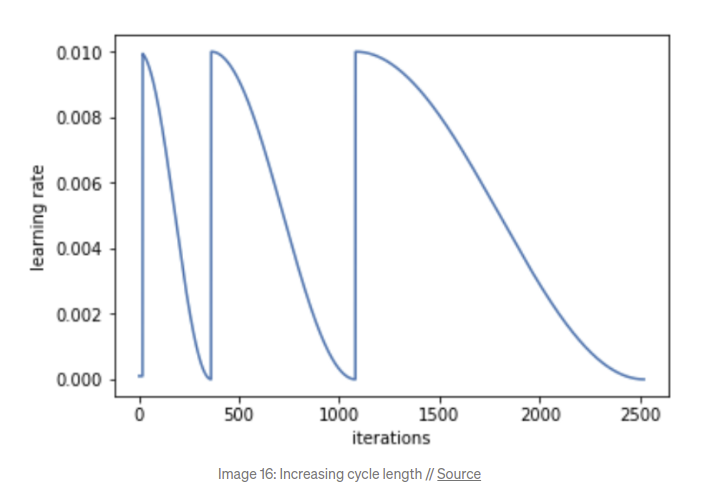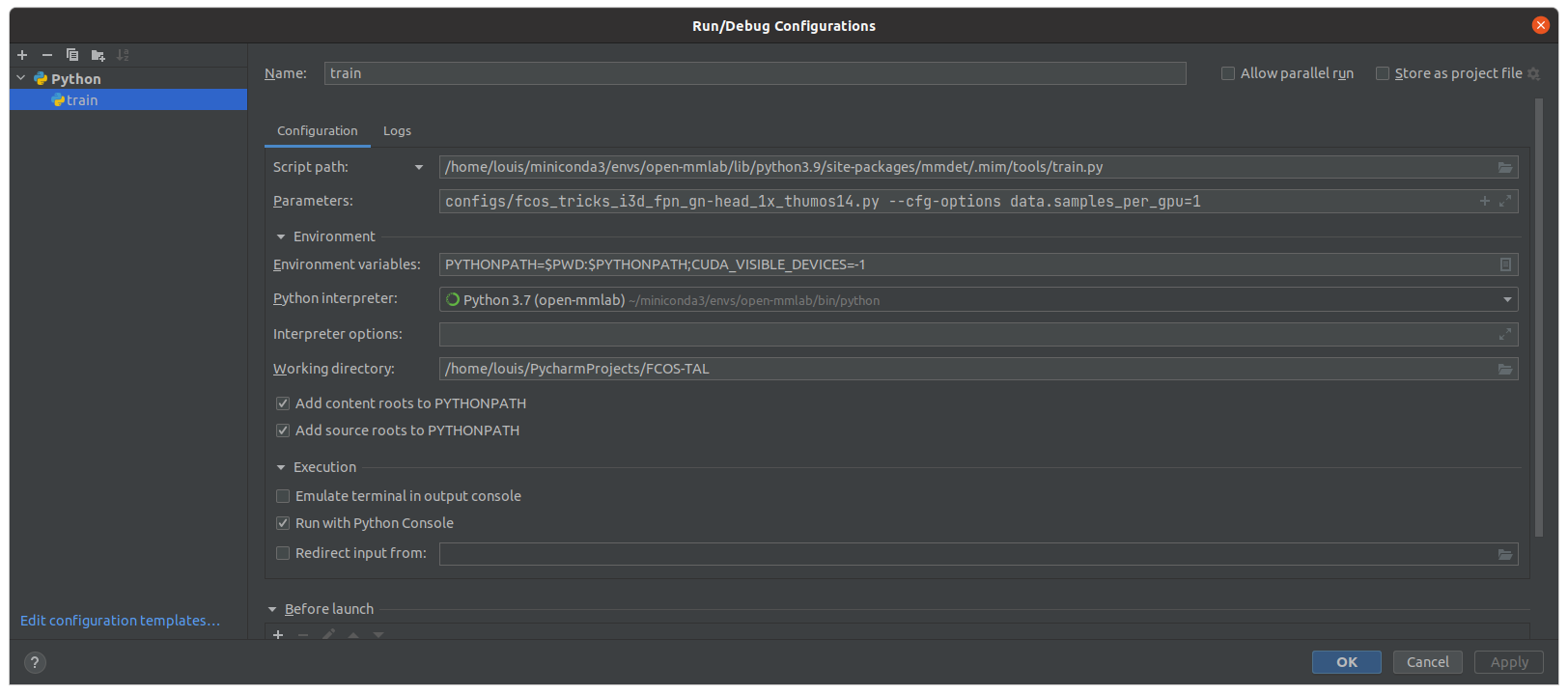# Basic config variables

Here we do NOT expalin the meaning of variables in the config, which we refer the reader to the official document. Instead, we go deep into the arguments of config variables, e.g. `by_epoch` of variables `evaluation`

## evalution

This hook will regularly perform evaluation on validation dataset in a given interval.

Example in config:

``````evaluation = dict(interval=5, metrics=['top_k_accuracy', 'mean_class_accuracy'])
``````

Arguments:

``````    start (int | None, optional): Evaluation starting epoch. It enables
evaluation before the training starts if ``start`` <= the
resuming epoch. If None, whether to evaluate is merely decided
by ``interval``. Default: None.
interval (int): Evaluation interval. Default: 1.
by_epoch (bool): Determine perform evaluation by epoch or by
iteration. If set to True, it will perform by epoch.
Otherwise, by iteration. default: True.
save_best (str | None, optional): If a metric is specified, it
would measure the best checkpoint during evaluation. The
information about best checkpoint would be save in best.json.
Options are the evaluation metrics to the test dataset. e.g.,
``top1_acc``, ``top5_acc``, ``mean_class_accuracy``,
``mean_average_precision``, ``mmit_mean_average_precision``
for action recognition dataset (RawframeDataset and
VideoDataset). ``AR@AN``, ``auc`` for action localization
dataset. (ActivityNetDataset). ``mAP@0.5IOU`` for
If ``save_best`` is ``auto``, the first key of the returned
``OrderedDict`` result will be used. Default: 'auto'.
rule (str | None, optional): Comparison rule for best score.
If set to None, it will infer a reasonable rule. Keys such as
'acc', 'top' .etc will be inferred by 'greater' rule. Keys
contain 'loss' will be inferred by 'less' rule. Options are
'greater', 'less', None. Default: None.
**eval_kwargs: Evaluation arguments fed into the evaluate function
of the dataset.
``````

Tips:

• By setting the `by_epoch=False`, we can conduct validation in a interval of iteration level. This can also be used to quicky enter to the validation phase for debugging purpose.
• The argumetns of function `Dataset.evaluate()` are set in here.
• `--resume-from work_dirs/foo/epoch_3.pth --cfg-options evaluation.interval=1 evaluation.start=3` can resume the training starting with a validation.
• `save_best='mAP', rule='greater'` or `save_best='loss', rule='less'` can help saving the best checkpoint based on a specific metric and rule.

## checkpoint_config

Save checkpoints periodically.

Example:

``````checkpoint_config = dict(interval=5)
``````

Arguments:

``````    interval (int): The saving period. If ``by_epoch=True``, interval
indicates epochs, otherwise it indicates iterations.
Default: -1, which means "never".
by_epoch (bool): Saving checkpoints by epoch or by iteration.
Default: True.
save_optimizer (bool): Whether to save optimizer state_dict in the
checkpoint. It is usually used for resuming experiments.
Default: True.
out_dir (str, optional): The directory to save checkpoints. If not
specified, ``runner.work_dir`` will be used by default.
max_keep_ckpts (int, optional): The maximum checkpoints to keep.
In some cases we want only the latest few checkpoints and would
like to delete old ones to save the disk space.
Default: -1, which means unlimited.
``````

Tips:

• Set `by_epoch=False` can save checkpoints by iterations.
• Set `max_keep_ckpts` to save disk space.

## log_config

Control the printed infomations and saved content in the .log file (`TextLoggerHook`). Other hooks like `TensorboardLoggerHook` can save specific logs.

Example:

``````log_config = dict(interval=20, hooks=[dict(type='TextLoggerHook'), dict(type='TensorboardLoggerHook')])
``````

LoggerHook Arguments:

``````    interval (int): Logging interval (every k iterations). Default 10.
ignore_last (bool): Ignore the log of last iterations in each epoch
if less than `interval`. Default True.
reset_flag (bool): Whether to clear the output buffer after logging.
Default False.
by_epoch (bool): Whether EpochBasedRunner is used. Default True.
**kwargs: Other arguments depends on the type of loggerhook.
``````

Tips:

• Enbale `TensorboardLoggerHook` is recommended. You can then use the command `tensorboad --logdir=\$path2work_dir` to visualize the training.
• only `interval` in `log_config` will be passed into every logger hook.
• `by_epoch=True` does NOT means log by epochs but indicting that EpochBasedRunner is used.
• The logged metrics are the average of the cumulative records in the last iters of one interval number   .

## img_norm_cfg

Normalize the magnitude of the image pixles `x = (x-mean) / std`.

``````    mean (Sequence[float]): Mean values of different channels.
std (Sequence[float]): Std values of different channels.
to_bgr (bool): Whether to convert channels from RGB to BGR.
Default: False.
on 'scale_factor' when modality is 'Flow'. Default: False.
``````

Example:

``````img_norm_cfg = dict(mean=[127.5, 127.5, 127.5], std=[127.5, 127.5, 127.5], to_bgr=False) # normalize to [-1, 1] without knowing statistics of dataset
``````
• `to_bgr` means whether to change the channel order from RGB to BGR. Normally False. Note that the mmaction2 decodes videos with `RGB` format by default.
• Kinetics400 norm config: `img_norm_cfg = dict(mean=[123.675, 116.28, 103.53], std=[58.395, 57.12, 57.375], to_bgr=False)` (used by the mmaction2)

## optimizer

Define the optimizer. In short, it points to the pytorch optimizers.

Example in config:

``````optimizer = dict(type='SGD', lr=0.01, momentum=0.9, weight_decay=0.0001)
``````
• Configure `paramwise_cfg` to set different learning rate for different model parts. For example, `paramwise_cfg=dict(custom_keys={'backbone': dict(lr_mult=0.1)})` will used `0.1*lr` for the backbone parameters.
Details In details, it [builds an optimizer-constructor](https://github.com/open-mmlab/mmcv/blob/00b003da230b5b9c42da634db09de537191941ea/mmcv/runner/optimizer/builder.py#L38), then the constructor [builds an optimizer from the registry](https://github.com/open-mmlab/mmcv/blob/00b003da230b5b9c42da634db09de537191941ea/mmcv/runner/optimizer/default_constructor.py#L243) and links the optimizer with model parameters. The [DefaultOptimizerConstructor](https://github.com/open-mmlab/mmcv/blob/00b003da230b5b9c42da634db09de537191941ea/mmcv/runner/optimizer/default_constructor.py#L13) is used by dafault and the only one available in `mmcv`. The DefaultOptimizerConstructor already supports some basic customization, e.g., different `lr` for `backbone` and `head`. If complext customization is needed, user may design their own optimizer-construtor to set different optimizer argumets, such as `lr`, `weight_decay`, for different model parameters. For example, the [TSMOptimizerConstructor](https://github.com/open-mmlab/mmaction2/blob/26a105b32666d0ea6e9e33d7dbef8affa936f099/mmaction/core/optimizer/tsm_optimizer_constructor.py#L8) in `mmaction2`. ### [DefaultOptimizerConstructor](https://github.com/open-mmlab/mmcv/blob/00b003da230b5b9c42da634db09de537191941ea/mmcv/runner/optimizer/default_constructor.py#L13) **Arguments**: ```python Positional fields are - `type`: class name of the optimizer. Optional fields are - any arguments of the corresponding optimizer type, e.g., lr, weight_decay, momentum, etc. paramwise_cfg (dict, optional): Parameter-wise options. ```

## optimizer_config

Define the optimizer hook. In short, it points to the torch.nn.utils.clip_grad_norm_.

Example:

``````optimizer_config = dict(grad_clip=dict(max_norm=40, norm_type=2))
``````
Details All available optimizer hooks can be found in [here](https://github.com/open-mmlab/mmcv/blob/22e73d69867b11b6e2c82e53cdd4385929d436f5/mmcv/runner/hooks/optimizer.py#L22). Some hooks are used for GradientCumulative and some are for the Fp16. ### [OptimizerHook](https://github.com/open-mmlab/mmcv/blob/22e73d69867b11b6e2c82e53cdd4385929d436f5/mmcv/runner/hooks/optimizer.py#L23) **Arguments:** ```python grad_clip=None, detect_anomalous_params=False ```

## fp16 = dict()

Comment/Uncomment`fp16 = dict()` in config to switch between the FP16 training. Before using it, you need decorate your model’s forward function with `@auto_fp16` and set a attribute `self.fp16_enable = False`. For example:

``````from mmcv.runner import auto_fp16

@RECOGNIZERS.register_module()
class AnyModel(nn.Module):
"""APN model framework."""

def __init__(self, ...):
...
self.fp16_enabled = False    # Default to False. If `fp16=dict()` in config, it will be automatically turn to True.

@auto_fp16()
def forward(self, x):
return self.conv(x)
``````

Results | | Model | Memory | Runtime | Accuracy | |——-|——-|——–|———|———-| | fp32 | MViT-B| 9036M | 76s | todo | | fp16 | | 8068M | 125s | todo |

# Meticulous config variables

## pipelines

### RandomResizedCrop

Random crop that specifics the area and height-weight ratio range of the cropped shape.

Arguments:

``````    area_range (Tuple[float]): The candidate area scales range of
output cropped images. Default: (0.08, 1.0).
aspect_ratio_range (Tuple[float]): The candidate aspect ratio range of
output cropped images. Default: (3 / 4, 4 / 3).
lazy (bool): Determine whether to apply lazy operation. Default: False.
``````

Tips:

• This data augmentation mimic the `torch.RandomResizedCrop`, which is widly used in image-based tasks, known as the Inception-style random cropping. It first determine the cropping shape, whose aspect ratio and area is randomly selected in the given range. Then with the specific cropping shape, it randomly crop a sub-area from the input.
• This pipeline is normally followed by a `Resize(scale=(224, 224), keep_ratio=False)`. Besides, it normally follows a `Rescale(scale=(-1, 256))`, i.e., rescale the short-side to 256. I suggest to conduct the short-side rescaling offline because it is fixed. Offline rescaling can reduce the data augmentation time and sometimes can significantly reduce the disk size used by the datasets, e.g., kinetics400.

### RandomShortSideScale

Scale that specifics the range short-side size.

Example

``````train_pipeline = [
dict(type='RandomRescale', scale_range=(256, 320)),
dict(type='RandomCrop', size=224),
]
val_pipeline = [
dict(type='Resize', scale=(-1, 256)),
dict(type='CenterCrop', crop_size=224),
]
test_pipeline = [
dict(type='Resize', scale=(-1, 256)),
dict(type='ThreeCrop', crop_size=256),
]
``````

Arguments:

``````    scale_range (tuple[int]): The range of short edge length. A closed
interval.
interpolation (str): Algorithm used for interpolation:
"nearest" | "bilinear". Default: "bilinear".
``````

Tips:

• Compared to the `RandomResizedCrop`, it scale the input volume’s short side to a randomly selected int from the given range.
• This pipeline is normally followed by a `RandomCrop(size=(224, 224))`.
• For these whose datasets are already rescaled to short-side=256, this pipeline is not a good choice.
• This data augmentation was used by the vgg, non-local, slowfast, x3d. However, the latest paper MViT2, from the same team of x3d and slowfast, now also used the Inception-style cropping, i.e. `RandomResizedCrop`, for data augmention in training.
• There is another pipeline which adopts the pytorchvideo lib, i.e., [PytorchVideoTrans(type=’RandomShortSideScale’)] , and it should perform the same.
• During inference, one can either scale the short side to 256 and take a 224 center crop, or scale the short side to 256 and take three 256 crops along longer side.

### SampleFrames and DenseSampleFrames

1. Let’s note the `clip_len`, `frame_interval`, and `num_clips` as (clip_len x frame_interval x clip_len), e.g. (16x4x2)
2. `SampleFrames(16x4x1)`: This is the most frequently used, and the most basic sampling strategy. It randomly samples 16 frames with interval 4 from the input video. It can be regarded as first randomly cropping a 64-frame sub-video from the input video, then uniformally sampling 16 frames from the cropped sub-video. The output shaoe is (16, H, W)
3. `SampleFrames(16x4x8)`: Now the `num_clips=8`, this can be regarded as first dividing the input video into 8 sub-videos, then conducting the same operations as the `SampleFrames(16x4x1)` on each sub-video. This example is just for explanation. I have never seen such a combiantion of arguments. The output shape is (256, H, W)
4. `SampleFrames(1x1x8)`: Same as the point 2, but in this case we only randomly sample one frame in each of the 8 sub-videos. This sampling strategy is what the TSN proposes to use to cover global information of the videos. And I found that TSN is the only one using this kind of arguments combination. The output shape is (8, H, W), whereas accoring to the TSN, each of the 8 frames will be seperately fed into a 2D ResNet, i.e., the 8 will be multiplied into the batch_size dimension.
5. `DenseSampleFrames(16x4x2)`: Similar to `SampleFrames`, but in this case it first crop a sub-video of length 64-frames (arg of `DenseSampleFrames` default=64) from the input video, then apply the `SampleFrames(16x4x2)` on the cropped sub-video. When the `num_clips=1`, `DenseSampleFrames` is same as the `SampleFrames`. This pipeline is hardly used in mmaction2 and I have never seen similar sampling strategy in the literatures. Maybe it’s a experimental pipeline.
6. `SampleFrames(16x4x10, test_mode=True)`: Uniformly dividing the input video to 10 clips; At the center of each clip, sampling 16 frames in interval 4. This is the most frequently used temporal sampling strategy during the inference, known as 10 views.

Arguments:

``````    clip_len (int): Frames of each sampled output clip.
frame_interval (int): Temporal interval of adjacent sampled frames.
Default: 1.
num_clips (int): Number of clips to be sampled. Default: 1.
temporal_jitter (bool): Whether to apply temporal jittering.
Default: False.
twice_sample (bool): Whether to use twice sample when testing.
If set to True, it will sample frames with and without fixed shift,
which is commonly used for testing in TSM model. Default: False.
out_of_bound_opt (str): The way to deal with out of bounds frame
indexes. Available options are 'loop', 'repeat_last'.
Default: 'loop'.
test_mode (bool): Store True when building test or validation dataset.
Default: False.
start_index (None): This argument is deprecated and moved to dataset
class (``BaseDataset``, ``VideoDatset``, ``RawframeDataset``, etc),
see this: https://github.com/open-mmlab/mmaction2/pull/89.
keep_tail_frames (bool): Whether to keep tail frames when sampling.
Default: False.
``````

Tips:

• If `test_mode=True`, then the cropping in each clip happens at the temporal center.
• Don’t worry about the arg `num_clips` (just set them to the defaul 1) unless you want to use sampling strategy like the TSN, TSM used.
• `out_of_bound_opt` is used to handle the occasion that the input video is shorter than `clip_len x frame_interval`. Normally it’s not important. I mention this just for better understanding.
• For arg `keep_tail_frames`, its motivation can be found in here. Don’t need care about it if `num_clips=1`

### ThreeCrop

Uniformly crops three crops along the longer side. Normally conducted on short-side rescaled inputs and the crop_size is equal to the short-side, e.g., `Resize(-1, 256)`-`ThreeCrop(crop_size=256)`.

Arguments:

``````    crop_size(int | tuple[int]): (w, h) of crop size.
``````

Tips:

• The `crop_size` normally is NOT equal to the training crop size. For example, x3d, slowfast, non-local all use the `Resize(-1, 256)`- `RandomResizedCrop`-`Resize(224, 224)` in the training, but the `Resize(-1, 256)`-`ThreeCrop(crop_size=256)` in the testing. It means the spatial size of the model input used in the test is larger (256 vs. 224) than the ones used in the traning. It won’t cause dimension error because of the AdaptivePool between the backbone and the head. Its technical reason can be found in the paper – Very Deep Convolutional Networks for Large-Scale Image Recognition. A complete config example can be found in here

### TenCrop

Spatially crop the images into 10 crops (8corner + 1center + 1flip). Normally share the same `Rescale` and `Resize` opreations with the training, e.g., `Resize(-1, 256)`-`ThreeCrop(crop_size=224)`.

Arguments:

``````    crop_size(int | tuple[int]): (w, h) of crop size.
``````

Tips:

• Better performance compared with `ThreeCrop` but higher computational complexity, sometimes even cause the OOM problem.

## lr_config

Configure how to update the learning rate. Constant lr will be used if no configuration. All lr_updater hooks

Example in config:

``````lr_config = dict(policy='step', step=[4, 8], gamma=0.1)  # lr = lr * 0.1 after epoch 4 and 8. No warmup used.
``````

### LrUpdaterHook

args:

``````by_epoch=True,
warmup=None,
warmup_iters=0,
warmup_ratio=0.1,
warmup_by_epoch=False
``````

### StepLrUpdaterHook

args:

``````step,
gamma=0.1,
min_lr=None
``````

### CosineAnnealingLrUpdaterHook

args:

``````min_lr=None,
min_lr_ratio=None
``````

This cosine-anneling does NOT include the restart, but it is more frequently used because it has less hyper-parameters.

### CosineRestartLrUpdaterHook

args:

``````periods,
restart_weights=,
min_lr=None,
min_lr_ratio=None
``````

This is the complete cosine-annealing of the original paper. But it’s less used.

I finally find that the formula turns to be the most simple way to understand the cosine annealing, which can be found in related pytorch pages:Unlike the pytorch that use the period of first restart `T_0` and the period multiplicative factor `T_mult` to control the lr, the `mmaction2` requires to specify the periods of all restarts. And it supports restarting with weights. For the above example, it has three consine restarts in its (5+10+15=30) epochs, the first two restarts begin with `base_lr` and end with `0.1*base_lr`, while the last restart begins with `0.5*base_lr` and end with `0.05*base_lr`. The weights is related to last restart.

# Miscellaneous

## `RawframeDecode` with beckend `pyturbo`

According to a issue in mmcv, using TurboJPEG instead of cv2 for RGB image decoding and increase the speed of image decoding about 3 times (I have not testified it yet).

Example:

``````...
dict(type='RawFrameDecode', decoding_backend='turbojpeg'),
...

``````

To install:

``````pip install PyTurboJPEG
``````

However, my `pip` installation of pyturbo has a problem:

``````RuntimeError: Unable to locate turbojpeg library automatically. You may specify the turbojpeg library path manually.
e.g. jpeg = TurboJPEG(lib_path)
``````

and I found a solution using the `sudo` installation:

``````sudo apt-get update -y
sudo apt-get install -y libturbojpeg
``````

## Debug `mmlab` with Pycharm

For simplicity of debugging, cpu is used and batch_size=1.## Fix random seed

### For mmlab repository without mmengine (old versions)

• in `--cfg-options`, adding `--seed=2 --deterministic --cfg-options data.videos_per_gpu=1`
• in config file, adding `torch.backends.cudnn.benchmark = True`
• in config file data setting, adding `train_dataloader=dict(shuffle=False)`

### For mmengine based mmlab repository

todo

`_load_checkpoint(filename)`load the model weights (state_dict) from the filename to a variable.
`_load_checkpoint_with_prefix(prefix, filename)`load the model weights from the filename, remove the prefix in the state_dict.
`load_checkpoint(model, filename)`load the model weights from the filename, and init the model with the loaded state_dict.
`load_state_dict(module, state_dict)`init the model with the state_dict.
`initialize(module, init_cfg)`initialize the model with init_cfg. For examples, `init_cfg=dict(type='Pretrained', checkpoint='torchvision://resnet50')`, `init_cfg=dict(type='Constant', val=1, bias=2, layer='Conv2d')`. More init class can be found in the mmcv.cnn.utils.weight_init.py
``````--cfg-options work_dir=work_dirs/test log_config.interval=1 evaluation.by_epoch=False evaluation.interval=5